# Plane Geometry

If you like drawing, then geometry is for you!Plane Geometry is about flat shapes like lines, circles and triangles ... shapes that can be drawn on a piece of paper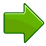Hint: Try drawing some of the shapes and angles as you learn ... it helps.

## Point, Line, Plane and Solid

A Point has no dimensions, only position
A Line is one-dimensional
A Plane is two dimensional (2D)
A Solid is three-dimensional (3D)

Plane Geometry is all about shapes on a flat surface (like on an endless piece of paper).### Polygons

A Polygon is a 2-dimensional shape made of straight lines. Triangles and Rectangles are polygons.

Here are some more:

### The Circle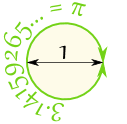Circle Theorems (Advanced Topic)

### Symbols

There are many special symbols used in Geometry. Here is a short reference for you:

Geometric Symbols

### Angles

Types of AnglesAcute Angles Right Angles Obtuse Angles Straight Angle Reflex Angles Full Rotation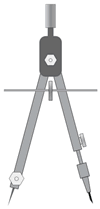### Using Drafting Tools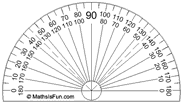### Transformations and Symmetry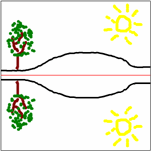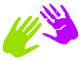## More Advanced Topics in Plane Geometry

### Pythagoras### Conic Sections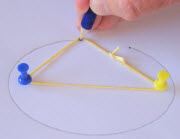## TrigonometryTrigonometry is a special subject of its own, so you might like to visit: